# Learn the concept of Object Oriented Programming in Python

1
3838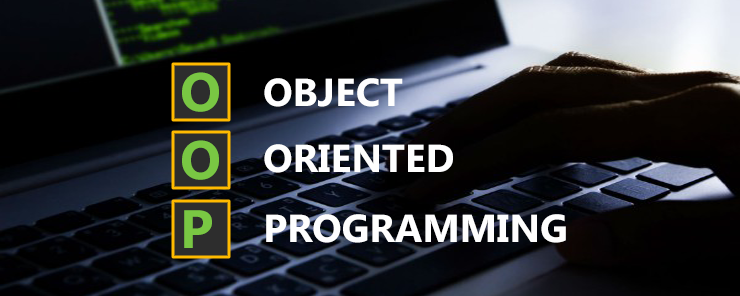Earlier in this series, I told you that one of the main features of the Python language is that it supports Object Oriented Programming.

Why OOP?
The fundamental idea behind object oriented programming is to combine into a single unit both data and the functions that operate on that data. Such unit is called an object. (Object Oriented Programming in C++ – Robert Lafore, © 1995).

So, the object (as Robert Lafore has told us) is a unit that contains both data (attributes), and functions (methods) that work on that data. That is the object, so what about the class? What does it mean? And what is the relation between objects and classes?

Well, people say object X is a member of class Y. This is analogous to saying the variable x is of the type Integer, and the variable firstname is of the type String. So, the relation between objects and classes is the same relation between variables and data types. Have you got the idea? Nice.

As variable can belong to one (and only one) data type (variable x can’t be an integer and string in the same time), an object can be a member of only one class. On the other hand, a class can have many instances (objects) that belong to it, exactly like many variables can be of the type string.

– Well, but you haven’t answered the question yet: Why OOP?

Okay, object oriented programming offers a number of benefits. The most important of these benefits are:

Encapsulation: Data (attributes) of an object are said to be encapsulated (hidden) inside this object. In other words, in most cases, the data are defined as private, so that the user has no direct access to it. This serves the security from two ways: the first, to prevent unauthorized read to the data. The second, to prevent accidental (or malicious) alteration of the object data. So, the encapsulation helps ensure both Confidentiality and Integrity of data.

Inheritance: Inheritance means a class definition can be extended (inherited) by another class. The new class can be considered as sub-class of the original class (which is now called superclass). To get the idea closer to your mind consider the following simple example (also from Robert Lafore): The class of vehicles is divided into cars, trucks, buses, and motorcycles.
Besides to inheriting the data and functions of the superclass, the subclass can add its own attributes and methods as well. This enriches the developing process.
Due to the great importance of this concept, a separate article will be dedicated to discuss it, with details.

Code Reusability: after being written, complied, and tested, a class can be distributed (published), so that other developers can use it (or extend it by inheritance). This also enriches the developing process, and helps the developers’ community cooperate and benefit from others’ work.

New Data Types: As a class to an object is like the data type to a variable; classes can be considered new user-defined data types. Consider a point in the plane. To locate the point, you need both x and y coordinates of that point. Both x and y are numeric data types. Now, consider defining a class called Point, which contains two attributes: the x coordinate and the y coordinate. This way, we have a new composite data type that contains the two member variables x and y.

Overloading: Overloading means the same function name (or the same operator) can be defined more than once, given that the numbers of arguments are different, or the types of the arguments are different between each function definition.

There are many other benefits for using the object oriented approach in programming. You will discover these benefits yourself by time.

* * * * * *

After this theoretical introduction, I bet your fingers are now touching the keyboard in reflexive motions, because they want to start typing code. Okay, let’s move into syntaxes.

The Class definition Syntax

```class class_name:
set_of_
methods_
and_
attributes
```

Example
The following code will define a class named MyClass. This class contains two methods, one for setting the value of the member variable data, and the other to get it.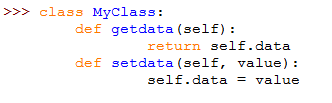Now, let’s see our first class in action. We are going to define two instances (objects) that belong to this class. This is done as follows: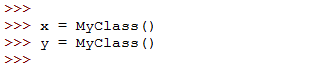To set the value of the member variable data, we will use the setdata method: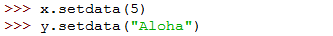Now, to retrieve the value stored in this member variable from both objects, the getdata method comes into light: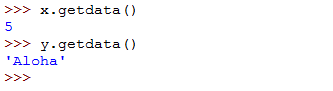The complete code for the example is as follows: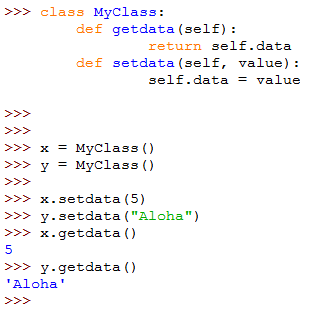* * * * * *

Example

Now, let’s implement the Point class that we mentioned earlier in this article (under the New Data Types feature).
Again, the point is defined by its two coordinates: x and y. I am certain you have guessed that our class is going to have two member variables: x and y. These member variables will need methods to set and get values for them.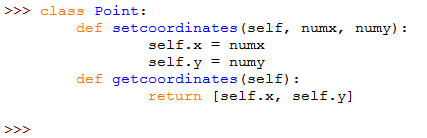Notice that in the member function getcoordinates, I have used a list of two elements (x and y) to be returned. This is because only one return statement can be executed in a function. Otherwise, I will have to create a separate function to get the value of each member variable (which is possible, but boring task, don’t you agree with me?!)
Now, let’s define an object of this class: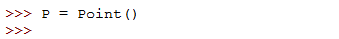To set the value of both coordinates, the setcoordinates member function is used: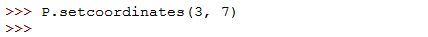To get the values of the x and y coordinates of the defined Point object P, the getcoordinates member function will be used. The values of x and y appear as the elements of the two-elements array returned from the get function.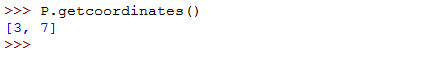The complete program is as follows: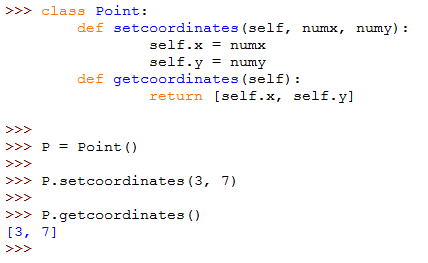* * * * * *

In this article, we have introduced the concept of object oriented programming. After showing the benefits of using OOP as a developing approach, we have presented the basic syntax for defining a class, and illustrated it with two examples.

1.meenal deshpande